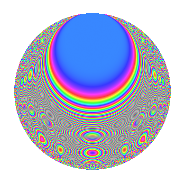# Properties

 Label 150.2.lLevel 150 Weight 2 Character orbit l Rep. character $$\chi_{150}(17,\cdot)$$ Character field $$\Q(\zeta_{20})$$ Dimension 80 Newforms 1 Sturm bound 60 Trace bound 0

# Related objects

## Defining parameters

 Level: $$N$$ = $$150 = 2 \cdot 3 \cdot 5^{2}$$ Weight: $$k$$ = $$2$$ Character orbit: $$[\chi]$$ = 150.l (of order $$20$$ and degree $$8$$) Character conductor: $$\operatorname{cond}(\chi)$$ = $$75$$ Character field: $$\Q(\zeta_{20})$$ Newforms: $$1$$ Sturm bound: $$60$$ Trace bound: $$0$$

## Dimensions

The following table gives the dimensions of various subspaces of $$M_{2}(150, [\chi])$$.

Total New Old
Modular forms 272 80 192
Cusp forms 208 80 128
Eisenstein series 64 0 64

## Trace form

 $$80q + 4q^{3} + 4q^{7} + O(q^{10})$$ $$80q + 4q^{3} + 4q^{7} - 4q^{10} - 4q^{12} - 8q^{15} + 20q^{16} - 8q^{18} - 40q^{19} - 36q^{22} - 104q^{25} + 4q^{27} - 16q^{28} + 12q^{30} + 4q^{33} - 40q^{34} - 24q^{37} - 40q^{39} - 8q^{40} - 4q^{42} - 24q^{43} - 72q^{45} - 4q^{48} - 12q^{55} - 64q^{57} + 20q^{58} + 24q^{60} + 64q^{63} + 96q^{67} + 140q^{69} + 76q^{70} + 8q^{72} + 100q^{73} + 132q^{75} + 100q^{78} + 80q^{79} - 40q^{81} + 96q^{82} + 60q^{84} + 32q^{85} + 80q^{87} + 4q^{88} + 52q^{90} + 12q^{93} - 32q^{97} + O(q^{100})$$

## Decomposition of $$S_{2}^{\mathrm{new}}(150, [\chi])$$ into irreducible Hecke orbits

Label Dim. $$A$$ Field CM Traces $q$-expansion
$$a_2$$ $$a_3$$ $$a_5$$ $$a_7$$
150.2.l.a $$80$$ $$1.198$$ None $$0$$ $$4$$ $$0$$ $$4$$

## Decomposition of $$S_{2}^{\mathrm{old}}(150, [\chi])$$ into lower level spaces

$$S_{2}^{\mathrm{old}}(150, [\chi]) \cong$$ $$S_{2}^{\mathrm{new}}(75, [\chi])$$$$^{\oplus 2}$$